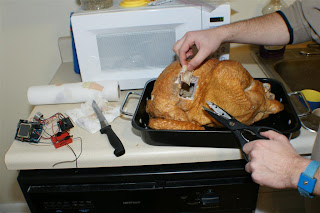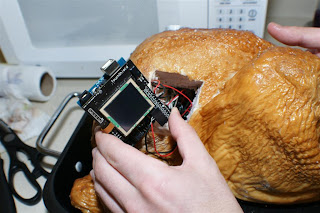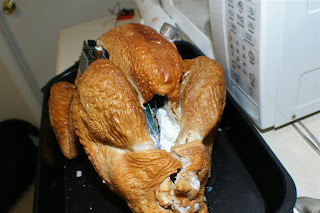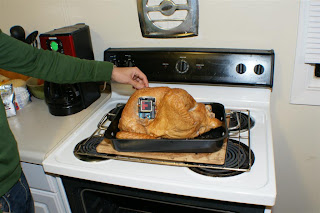## Wednesday, November 26, 2008

### Introducing the 8-bit embedded TurkeyShield

I'm always looking out for new ways to add more shields and components to my Arduino, and this felt like a natural (and festive) experiment, so I gave it a shot: it's an Arduino, Potentiometer (for user input), accelerometer (to know it's bearings), and compass (so the turkey's always facing due north), Lithium Backpack (for mobility of course), and TouchShield Stealth (for output) wired through a fairly large turkey :)

I've just uploaded all the pictures over here on Flickr.

In the DIY spirit, of course, here's a 5-step guide to making your own 8-bit embedded turkey:

1) Buy and cook a turkey (thanks for the help, Mike)2) Cut a square hole in one side for the TouchShield, and a small round hole in the other side for the potentiometer3) Insert the potentiometer knob and TouchShield into the turkey (trying carefully not to short any exposed wires through the turkey, since it's technically conductive):I didn't believe it either, but I tested it: turkeys are conductive - this is why I had to do the next step:4) Place the Lithium Backpack in a plastic baggie, with the wires coming out the top. It's a little risky putting an exposed lithium battery cell up against conductive turkey meat, so this is an important step:5) Upload program code to read the accelerometer and compass from Parallax:Of course it's open source, so the code is pasted at the bottom of the post

Here's a video of the full "circuit" in action:

Here's the source code to run on the Arduino (thanks, kiilo!):

#include

#define RXPIN 3
#define TXPIN 2

AFSoftSerial mySerial = AFSoftSerial(RXPIN, TXPIN);

unsigned char x=0;

/*
/////////////////////////////////
Htachi HM55B Compass
parallax (#)

AUTHOR: kiilo kiilo@kiilo.org

http://parallax.com/Store/Microcontrollers/BASICStampModules/tabid/134/txtSearch/hm55b/List/1/ProductID/98/Default.aspx?SortField=ProductName%2cProductName
http://sage.medienkunst.ch/tiki-index.php?page=HowTo_Arduino_Parallax_HM55B_Kompass
http://arduino.cc/playground/HM55B

/////////////////////////////////
*/
#include // (no semicolon)
//// VARS
byte CLK_pin = 5;
byte EN_pin = 4;
byte DIO_pin = 6;

int X_Data = 0;
int Y_Data = 0;
long angle;

//// FUNCTIONS

void ShiftOut(int Value, int BitsCount) {
for(int i = BitsCount; i >= 0; i--) {
digitalWrite(CLK_pin, LOW);
if ((Value & 1 << i) == ( 1 << i)) {
digitalWrite(DIO_pin, HIGH);
//Serial.print("1");
}
else {
digitalWrite(DIO_pin, LOW);
//Serial.print("0");
}
digitalWrite(CLK_pin, HIGH);
delayMicroseconds(1);
}
//Serial.print(" ");
}

int ShiftIn(int BitsCount) {
int ShiftIn_result;
ShiftIn_result = 0;
pinMode(DIO_pin, INPUT);
for(int i = BitsCount; i >= 0; i--) {
digitalWrite(CLK_pin, HIGH);
delayMicroseconds(1);
ShiftIn_result = (ShiftIn_result << 1) + 1;
//Serial.print("x");
}
else {
ShiftIn_result = (ShiftIn_result << 1) + 0;
//Serial.print("_");
}
digitalWrite(CLK_pin, LOW);
delayMicroseconds(1);
}
//Serial.print(":");

// below is difficult to understand:
// if bit 11 is Set the value is negative
// the representation of negative values you
// have to add B11111000 in the upper Byte of
// the integer.
// see: http://en.wikipedia.org/wiki/Two%27s_complement
if ((ShiftIn_result & 1 << 11) == 1 << 11) {
ShiftIn_result = (B11111000 << 8) | ShiftIn_result;
}

return ShiftIn_result;
}

void HM55B_Reset() {
pinMode(DIO_pin, OUTPUT);
digitalWrite(EN_pin, LOW);
ShiftOut(B0000, 3);
digitalWrite(EN_pin, HIGH);
}

void HM55B_StartMeasurementCommand() {
pinMode(DIO_pin, OUTPUT);
digitalWrite(EN_pin, LOW);
ShiftOut(B1000, 3);
digitalWrite(EN_pin, HIGH);
}

int result = 0;
pinMode(DIO_pin, OUTPUT);
digitalWrite(EN_pin, LOW);
ShiftOut(B1100, 3);
result = ShiftIn(3);
return result;
}

//Pin definitions
int xaccPin = 7;
int yaccPin = 8;

//Global variables
long xacc = 0;
long yacc = 0;

int count = 0;
long accel = 0;
int value = 0;

while(value == HIGH) { // Loop until pin reads a low
}

while(value == LOW) { // Loop until pin reads a high
}

while(value == HIGH) { // Loop until pin reads a low and count
count = count + 1;
}

//accel = abs(8 * (count * 18 / 10 - 500)); //this was the original function
accel = count;

return accel;
}

void setup() {
Serial.begin(9600);
pinMode(EN_pin, OUTPUT);
pinMode(CLK_pin, OUTPUT);
pinMode(DIO_pin, INPUT);
pinMode(xaccPin, INPUT);
pinMode(yaccPin, INPUT);

HM55B_Reset();

mySerial.begin(9600);

}

unsigned int a, xa, ya;

void loop() {
HM55B_StartMeasurementCommand(); // necessary!!
delay(40); // the data is 40ms later ready
//Serial.print(" ");
X_Data = ShiftIn(11); // Field strength in X
Y_Data = ShiftIn(11); // and Y direction
//Serial.print(X_Data); // print X strength
//Serial.print(" ");
//Serial.print(Y_Data); // print Y strength
//Serial.print(" ");
digitalWrite(EN_pin, HIGH); // ok deselect chip
angle = 180+ (180 * (atan2(-1 * Y_Data , X_Data) / M_PI)); // angle is atan( -y/x) !!!

a = angle;
xa = xacc;
ya = yacc;

//Print the output to the serial port:
Serial.print(a); // print angle
Serial.print(" ");
Serial.print(xa);
Serial.print(" ");
Serial.print(ya);
Serial.println("");

x=0;
while (x < 6)
{
serial_sendAnalog(x);
x++;
}

delay(10);

x=0;
while(x< 14)
{
serial_sendDigital(x);
x++;
}

delay(100);
}

void serial_sendDigital(unsigned char digitalPin)
{

if ( (digitalPin <> 13) )
return;

mySerial.print((unsigned char)1);
delay(2);

}

void serial_sendAnalog(unsigned char analogPin)
{
unsigned char lowByte, highByte;
unsigned int val;

/* Pin number range check */
if (analogPin > 6)
return;

/* Get the value */
val =10;
if (analogPin ==1) {
val = (2.5*a);
}
if (analogPin ==2) {
val = xa-700;
}
if (analogPin ==3) {
val = ya-700;
}
if (analogPin ==0) {
}
if (analogPin ==4) {
}
if (analogPin ==5) {
}

/* Separate the value into 2 bytes */
lowByte = (unsigned char)val;
highByte = (unsigned char)(val >> 8);

/* Send the high byte */
mySerial.print(highByte);

/* Write delay */
delay(1);

/* Send the low byte */
mySerial.print(lowByte);

/* Write delay */
delay(1);
}

And here's the code that runs on the TouchShield:

COLOR green = { 0, 255, 0 };
COLOR blue = {0,0,255};
COLOR yellow = {255,255,0};
COLOR BLACK = {0,0,0};
COLOR grey = {0x77,0x77,0x77};
COLOR red = {255,0,0};
COLOR black = {0,0,0};

unsigned int analogValues;
unsigned char digitalValues;

unsigned char x;

void setup()
{
drawBackground();

delay(8000);
Serial.begin(9600);

/* The sync character */
Serial.print('U');
}

void drawBackground()
{

}

void loop()
{

/* Read the analog values */
for (x=0; x< 6; x++)

for (x=0; x< 10; x++)

/* Redraw the analog values */
updateAnalog();
updateDigital();
}

void updateDigital()
{
unsigned char x;
char out;

green.red = 0;
green.green = 255;

for(x=0; x<10; x++)
{
//if (digitalValues[x])
// lcd_puts("H", digitalRect.left+2, digitalRect.bottom-8-(x*10), red, BLACK);
//else
// lcd_puts("L", digitalRect.left+2, digitalRect.bottom-8-(x*10), green, BLACK);
}
}

void updateAnalog()
{
unsigned char x;
char out;
float f;

f = ((float)analogValues/1024.0) * 120.0;
f = ((float)analogValues/1024.0) * 120.0;
f = ((float)analogValues/1024.0) * 120.0;
f = ((float)analogValues/1024.0) * 120.0;
f = ((float)analogValues/1024.0) * 120.0;
f = ((float)analogValues/1024.0) * 120.0;

/*
f = 64.0;
f = 64.0;
f = 64.0;
f = 64.0;
f = 64.0;
*/

//lcd_clearScreen( black);

drawhbar(1,10+19*0, 14, 120, (int)f);
drawhbar(1,10+19*1, 14, 120, (int)f);
drawhbar(1,10+19*2, 14, 120, (int)f);
drawhbar(1,10+19*3, 14, 120, (int)f);
drawhbar(1,10+19*4, 14, 120, (int)f);
drawhbar(1,10+19*5, 14, 120, (int)f);

/*
//lcd_rectangle(1,120-(int)f,1+14,120, red, red);
//lcd_rectangle(1,1,1+14,120-(int)f, black, black);
drawvbar(10+19*0, 120, 120, 14, (int)f);
drawvbar(10+19*1, 120, 120, 14, (int)f);
drawvbar(10+19*2, 120, 120, 14, (int)f);
drawvbar(10+19*3, 120, 120, 14, (int)f);
drawvbar(10+19*4, 120, 120, 14, (int)f);
drawvbar(10+19*5, 120, 120, 14, (int)f);
*/

for(x=0; x<6; x++)
{
//lcd_rectangle(1,2+12*x,f[x],12+12*x, red, red);
//lcd_rectangle(f[x],2+12*x,120,12+12*x, black, black);
//drawhbar(1,12*x, 12, 128, (int)f[x]);

//dtostrf(f[x],4,3,out);
//out=0;
//lcd_puts(out, 10, 10+(x*10), green, BLACK);

delay(10);
}

}

void drawhbar(int x, int y, int h, int w, int val) {
lcd_rectangle(x,y,val,y+h, red, red);
lcd_rectangle(x+val,y,w,y+h, black, black);
}

void drawvbar(int x, int y, int h, int w, int val) {
lcd_rectangle(x,y-val,x+w,y, red, red);
lcd_rectangle(x,y-h,x+w,y-val, black, black);
}